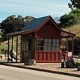# Gas station without pumps

## 2013 July 8

### 3-op-amp instrumentation amp

Filed under: Circuits course — gasstationwithoutpumps @ 15:03
Tags: , , ,

Yesterday in 2-op-amp instrumentation amp, I worked through the analysis of the differential gain and common-mode gain for an instrumentation amp made from two op amps.  There is a classic design of an instrumentation amp made from 3 op amps, and I was wondering how it compared in performance.  Both instrumentation amp designs are available as fairly low cost integrated circuits, but the ones with better common-mode rejection seem to use the 3-op-amp design.  Is there are reason for that?3-op-amp instrumentation amp. Schematic drawn with SchemeIt (using PNG export rather than a screenshot, since I have no special characters).

The 3-op-amp instrumentation amplifier consists of two separately analyzable parts. The first stage consists of two op amps that provide high-impedance inputs and amplify the differential signal without changing the common-mode signal. The second stage is a differential amplifier that zeros out the common-mode signal and provides unity gain on the differential signal.

We can analyze the first stage by setting the currents through the resistors equal:$(V_{a} - V_{m}) /R_{1} = (V_{p}-V_{b})/R_{2} = (V_{p} - V_{m})/R_{gain}$.
If we solve this for Va and Vb, we get$V_{a} = V_{m} - \frac{R_{1}}{R_{gain}} (V_{p} - V_{m})$$V_{b} = V_{p} + \frac{R_{2}}{R_{gain}} (V_{p} - V_{m})$

If we define$V_{comm} = \frac{V_{p} + V_{m}}{2} - V_{ref}$ and$\Delta V= V_{p}-V_{m}$, we can rewrite the output of the first stage as$V_{a} = V_{comm} + V_{ref} - (\frac{1}{2} +\frac{R_{1}}{R_{gain}}) \Delta V$$V_{b} = V_{comm} + V_{ref} + (\frac{1}{2} +\frac{R_{2}}{R_{gain}}) \Delta V$

The differential output of the first stage depends only on the differential input:$V_{b}-V_{a} = (1+ \frac{R_{1}+R_{2}}{R_{gain}}) \Delta V$

It may seem strange that I defined the common voltage so that I have an extra Vref floating around in the formula, but that simplifies out in the analysis of the second stage (which would not be the case if I had defined Vcomm as the just the average of Vp and Vm).

The differential amplifier that is the second stage can be described in terms of the two voltage dividers:$\frac{R_{4} V_{out} + R_{3} V_{a}}{R_{3} + R_{4}} = \frac{R_{5} V_{ref} + R_{6}V_{b}}{R_{5}+R_{6}}$.
Solving this for Vout gives us$V_{out} =\frac{V_{ref} R_{5} (R_{3}+R_{4}) + V_{b} R_{6} (R_{3}+R_{4}) - V_{a} R_{3} (R_{5}+R_{6})}{R_4 (R_{5}+R_{6})}$

If we substitute in the values of Va and Vb (and use Maple plus some hand algebra), we can simplify to$V_{out} - V_{ref} = \frac{1}{R_4 (R_{5}+R_{6})}\left((R_{4} R_{6} - R_{3}R_{5}) V_{comm} + \left(\frac{1}{2}(R_{3}R_{5} + R_{4}R_{6}) + R_{3}R_{6} + \frac{1}{R_{gain}}(R_{1}R_{3}(R_{5}+R_{6}) + R_{2}R_{6}(R_{3}+R_{4}))\right) \Delta V\right)$.

At R3=R4 and R5=R6, we can simplify to$V_{out} - V_{ref} = \frac{1}{2 R_{3}R_{5}} \left(0 V_{comm} + \left(2 R_{3}R_{5} + \frac{2 R_{3} R_{5} (R_{1}+R_{2})}{R_{gain}}\right)\Delta V\right)$,

which is$V_{out} - V_{ref} = \left(1+ \frac{R_{1}+R_{2}}{R_{gain}}\right)\Delta V$.  (Note: this result does not require that R1=R2 nor that R4=R5, though instrumentation amps are usually designed with those additional constraints, perhaps to maximize the range of acceptable input values, which this ideal-op-amp analysis ignores.)

The worst-case common-mode gain with 1% errors in the resistors would be with R4 and R6 high, and R3 and R5 low, giving a gain of 0.02 (or vice versa, for a common-mode gain of –0.02).  Comparing this to the 2-op-amp design’s common-mode gain of 0.04, we see that we gain a factor of 2 (6dB) in common-mode rejection by using an extra op amp.

1.[…] wiring to the electrodes.  With 130mV peak-to-peak common-mode voltage and a common-mode gain of 0.02 or 0.04, we’d have 2.6–5.2 mV of common-mode noise at the output of an instrumentation amp […]

Pingback by Common-mode noise in EKG | Gas station without pumps — 2013 July 8 @ 18:00

2.[…] resistors was unlikely to be reliable.  I discovered (after doing calculations for 2-op-amp and 3-op-amp designs) that 1% tolerance on the resistors would produce poor common-mode rejection. In […]

Pingback by Some failed designs | Gas station without pumps — 2013 July 10 @ 12:23

This site uses Akismet to reduce spam. Learn how your comment data is processed.